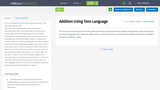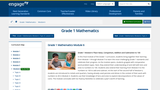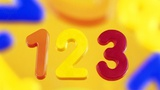# Search Results (5)

View
Selected filters:
• OnesUnrestricted Use
CC BY
Rating

This is a modification of the Illustrative Math lesson, "Ford and Logan ...

This is a modification of the Illustrative Math lesson, "Ford and Logan add 45 + 36," https://www.illustrativemathematics.org/content-standards/tasks/2068. This lesson was used with a tier 3 fourth grade intervention math group to help students develop their understanding of place value and use of tens language when adding two digit numbers. I modified it to meet the students' needs by adding ways two other students (Sarah and Tammy) solved the problem: Sarah said, "I know 4 tens plus 3 tens is 7 tens; 5 ones plus 6 ones is eleven ones which is 1 ten and 1 one so 4 tens plus 3 tens plus 1 tens is 8 tens and the one left so the answer is 81." Tammy said, " 45 plus 30 is 75, plus 6 more is 81." Students analyzed and discussed all four methods and compared them to their own methods. I then had students practice using the tens and ones language (like Sarah) for some more addition problems without using place value blocks or models.

Subject:
Mathematics
Material Type:
Author:
MEGAN HINDE
08/02/2019Conditional Remix & Share Permitted
CC BY-NC-SA
Rating

In this final module of the Grade 1 curriculum, students bring together ...

In this final module of the Grade 1 curriculum, students bring together their learning from Module 1 through Module 5 to learn the most challenging Grade 1 standards and celebrate their progress. As the module opens, students grapple with comparative word problem types. Next, they extend their understanding of and skill with tens and ones to numbers to 100. Students also extend their learning from Module 4 to the numbers to 100 to add and subtract. At the start of the second half of Module 6, students are introduced to nickels and quarters, having already used pennies and dimes in the context of their work with numbers to 40 in Module 4. Students use their knowledge of tens and ones to explore decompositions of the values of coins. The module concludes with fun fluency festivities to celebrate a year's worth of learning.

Subject:
Numbers and Operations
Material Type:
Module
Provider:
New York State Education Department
Provider Set:
EngageNY
12/30/2013Conditional Remix & Share Permitted
CC BY-NC-SA
Rating

Up to this point in Grade K, students have worked intensively within ...

Up to this point in Grade K, students have worked intensively within 10 and have often counted to 30 using the Rekenrek during fluency practice. This work sets the stage for this module where students clarify the meaning of the 10 ones and some ones within a teen number and extend that understanding to count to 100.

Subject:
Numbers and Operations
Material Type:
Module
Provider:
New York State Education Department
Provider Set:
EngageNY
02/05/2013Unrestricted Use
CC BY
Rating

This lesson was used with a tier 3 fourth grade intervention math ...

This lesson was used with a tier 3 fourth grade intervention math group to help students to progress from counting by tens and ones to combining and separating by tens and ones without skip counting. I used this Illustrative Math lesson, https://www.illustrativemathematics.org/content-standards/tasks/2106, and modified it to meet the students' needs. I modified the lesson by doing only the activities in Part 3 and then extending this by modeling the equations, that matched the hundreds chart work, with base ten blocks. I also made sure students saw the pattern in adding ones and adding tens and encouraged the proper place value terms. For example, "4 + 3 = 7 so 4 groups of ten + 3 groups of ten = 7 groups of ten which is the same as 40 + 30 = 70." I then extended this by having students add numbers with tens and ones to number with tens and ones with and without regrouping and asked them to first model it on the hundreds chart and with base ten blocks and then do the addition without skip counting (first adding the tens and then the ones and then combine the results).

Subject:
Mathematics
Material Type:
Author:
MEGAN HINDE
08/02/2019Conditional Remix & Share Permitted
CC BY-NC-SA
Rating

This lesson is for students who need support counting objects by ones. ...

This lesson is for students who need support counting objects by ones. This would be a helpful lesson for kindergarten students, or first grade students in need of additional practice with this skill.

Subject:
Mathematics
Material Type:
Lesson Plan
Author:
TARA OLK